# Text, Bytes and Videotape

January 2003

## Understanding bits and bytes

With 8 bits, we have 28= 256 possibilities (each bit can be zero or one, and the possibilities multiply together). We call 8 bits a byte. The very common ASCII system makes each letter of the alphabet, both capital and small (plus punctuation and some other symbols) correspond to a number from 0 to 255 (for example a=97, b= 98 and so on), so one letter can be expressed with one byte.

Thus the word "Shannon" takes 7 bytes. A page of a book (with 50 characters per line, 50 lines per page) takes 2,500 bytes, so a 400 page book takes 1,000,000 bytes. As with metres and kilometres, we need some new units, or we'd need a lot of zeroes! Hence we write that a page is approximately 2.5KB, and a book is approximately 1 MB (something strange happens here in that KB ("kilobyte") = 1,024 = 210 bytes, not 1000 as you might expect). It's easiest to keep track of things with powers of 2 like this. The next largest unit is 1MB ("megabyte") = 1,048,576 = 220 bytes, and then 1GB ("gigabyte") = 230 bytes.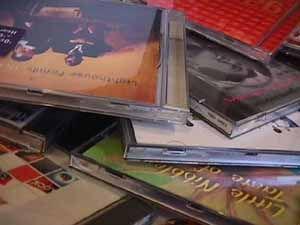Bits and bytes
Image from DHD photo gallery

Computers often represent a pixel of colour with 3 bytes. This is like mixing paint - the amount of red in the mixture varies from 0 to 255, the amount of green varies from 0 to 255, the amount of blue from 0 to 255. A computer monitor showing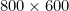pixels therefore needsbytes - more than a whole book - just to fill the screen. A picture really is worth more than a thousand words!

As for a movie, we need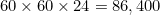pictures per hour (60 minutes per hour, 60 seconds per minute, 24 frames per second). That is, we’d need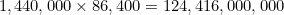bytes or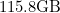to store an hour of film.

A CD-R can typically store about 700MB, a DVD can store about 4GB. It's clear we have to do something clever (that is, data compression) to fit a movie on a disc.

Back to main article

• Want facts and want them fast? Our Maths in a minute series explores key mathematical concepts in just a few words.